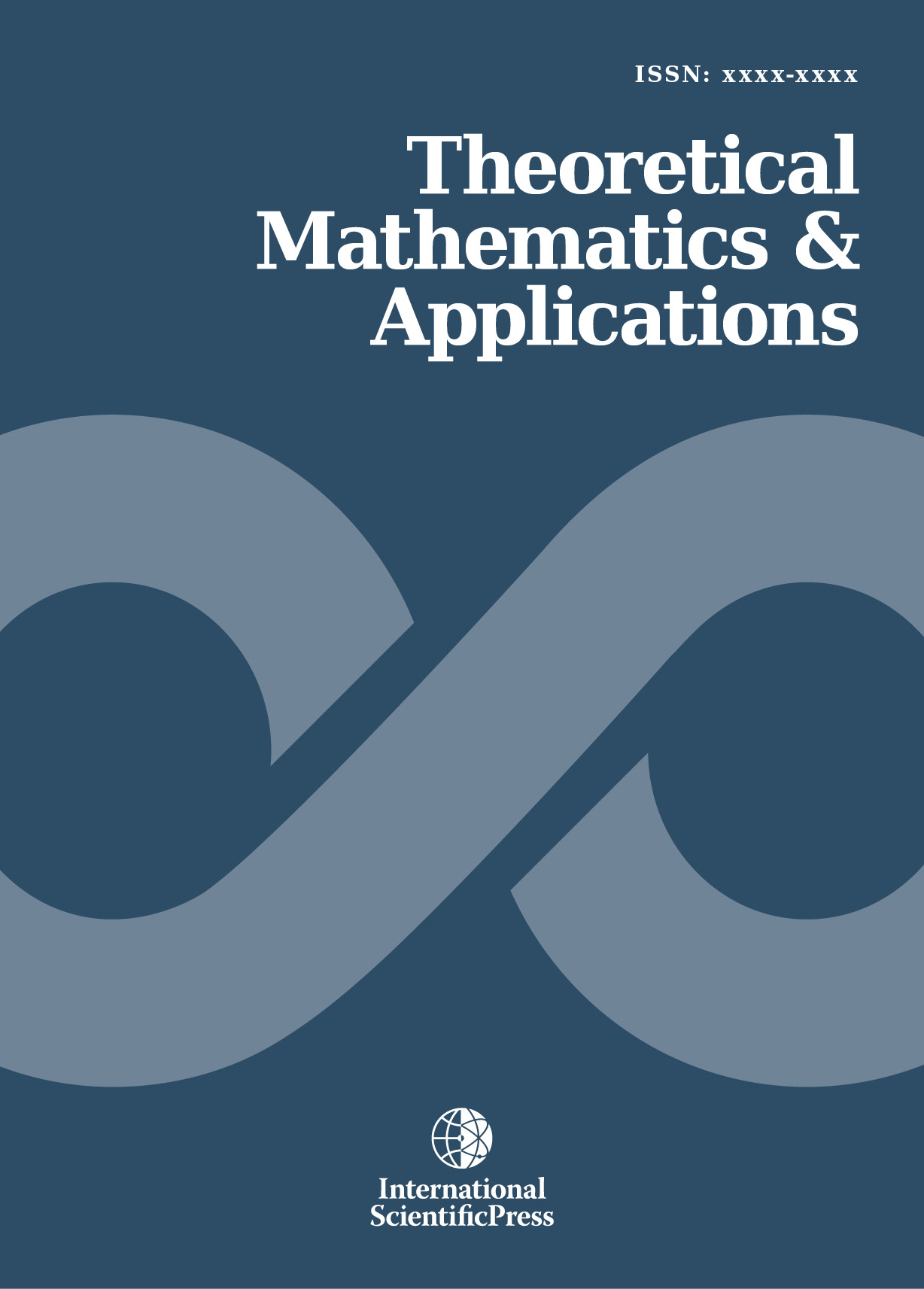# Theoretical Mathematics & Applications

#### Probability Distribution of Testing Time of the Software Based on Markov Chains

•[ Download ]
• Times downloaded: 1843
• Abstract

This Probability distribution of testing time of the software package is a problem waiting to be solved quickly. We transfer it into settling a series of problems which related to continuous-time Markov chains. Firstly, we construct a Q-matrix corresponding with the Markov chain X(t). Secondly, we solve the Kolmogorov forward equation corresponding with the Q-matrix and obtain the transition function Pij (t) of the Markov chain X(t). Finally, we get the probability distribution, expectation and variance of testing time of the Software.ISSN: 1792-9687 (Print)
1792-9709 (Online)